# An Intro to Wavelets for Computer Musicians

I wasn’t able to get this post fully complete in time for my self-imposed monthly deadline. I have decided to put it up in an incomplete state and clean it up in early September. I hope it is informative even in its current condition, which gets increasingly sketchy towards the end. Open during construction.

Among DSP types, those unfamiliar with wavelets often view them as a mysterious dark art, vaguely rumored to be “superior” to FFT in some way but for reasons not well understood. Computer musicians with a penchant for unusual and bizarre DSP (for instance, people who read niche blogs devoted to the topic) tend to get particularly excited about wavelets purely for their novelty. Is the phase vocoder too passé for you? Are you on some kind of Baudelairean hedonic treadmill where even the most eldritch Composers Desktop Project commands bore you?

Well, here it is: my introduction to wavelets, specifically written for those with a background in audio signal processing. I’ve been writing this post on and off for most of 2023, and while I am in no way a wavelet expert, I finally feel ready to explain them. I’ve found that a lot of wavelet resources are far too detailed, containing information mainly useful to people wishing to invent new wavelets rather than people who just want to implement and use them. After you peel back those layers, wavelets are surprisingly not so scary! Maybe not easy, but I do think it’s possible to explain wavelets in an accessible and pragmatic way. The goal here is not to turn you into a wavelet guru, but to impart basic working knowledge (with some theory to act as a springboard to more comprehensive resources).

Before we go further, I have to emphasize an important fact: while wavelets have found many practical uses in image processing and especially biomedical signal processing, wavelets are not that common in audio. I’m not aware of any widely adopted and publicly documented audio compression codec that makes use of wavelets. For both analysis alone and analysis-resynthesis, the short-time Fourier transform and the phase vocoder are the gold standard. The tradeoffs between time and frequency resolution are generally addressable with multiresolution variants of the STFT.

When wavelet transforms are used in audio, they are much more often used for analysis than for the implementation of wavelet-domain effects. Of course, commercial interests play a role here — there’s a lot more money in audio data mining than left-field musical effects — but fundamentally the Continuous Wavelet Transform is inherently difficult to use in effect design.

There is no one “wavelet transform” but a huge family of methods. New ones are developed all the time. To limit the scope of this post, I will introduce the two “classical” wavelet transforms: the Continuous Wavelet Transform (CWT) and Multiresolution Analysis (MRA). I’ll also go over popular choices of individual wavelets and summarize their properties. There are other wavelet transforms, some of more musically fertile than CWT or MRA, but you can’t skip the fundamentals before moving on to those. My hope is that demystifying wavelet basics will empower more DSP-savvy artists to learn about these curious creatures.

## Preliminaries

I should probably address a question some of my readers have: what are wavelet transforms? The best answer I can give is that a wavelet transform is any transform vaguely related to the original Continuous Wavelet Transform (CWT). While the Fourier transform takes a time-domain signal and converts it to frequency information, wavelet transforms capture both time and frequency. The short-time Fourier transform (windowed Fourier transform) does this too, but differently. Wavelet transforms are not inherently better than Fourier transform variants; they have their own advantages and disadvantages.

Wavelets are not as hard to grasp as some sources may make them seem, but I still wouldn’t jump into them if you’re a DSP beginner. You will need the following:

• the mathematics of the Fourier transform, which implies a grasp of complex numbers and single-variable integral calculus

• the convolution theorem, and the equivalence of linear time-invariant filters and convolution

• the Shannon–Nyquist sampling theorem

• what FIR filters are and how to implement them at a basic level (knowledge of classical filter design methods such as Parks–McClellan, etc. is not required)

I have papered over some finer mathematical details that are not necessary for working knowledge of wavelets. For example, the mathematician’s conception of $$L^2(\mathbb{R})$$ requires that functions that differ by finitely many points are considered equivalent. However, this distinction is unimportant for scientific and engineering applications. If you’re pedantic enough to care about that sort of thing, you’d be better off reading a proper wavelet textbook. Kaiser’s A Friendly Guide to Wavelets [Kaiser1994] is a good introduction (although “friendly” only to those with a math background), while Mallat’s A Wavelet Tour of Signal Processing [Mallat1999] is the definitive 800-page tome, a perfect holiday gift for the true wavelet lover in your life.

Any mistakes are my own. Get in touch if you find something wrong. Due to the size of this post [TODO: and the fact that it’s incomplete], errors are inevitable.

## Crash course on Hilbert spaces

A lot of resources on wavelets dive into operator theory and Hilbert spaces. We’ll take a small bite out of that field, only what we need for one-dimensional wavelets. If you want to dig deeper, the first chapter of [Kaiser1994] is pretty comprehensive.

The dot product of two $$n$$-dimensional vectors in $$\mathbb{R}^n$$ is given as:

\begin{equation*} x \cdot y = \sum_{i=1}^{n} x[i] y[i] \end{equation*}

The intuition for the dot product is that it produces a scalar that factors in both the similarity and lengths of the vectors. The dot product is related to the Euclidean norm:

\begin{equation*} ||x|| = \sqrt{x \cdot x} = \sqrt{\sum_{i=1}^n x[i]^2} \end{equation*}

The foundations of operator theory generalize these two concepts. The first step is to make the entries of the vectors generally complex-valued. An immediate hitch is that if we use the standard dot product, then in general $$x \cdot x$$ is no longer a nonnegative real number, and $$||x||$$ can’t be real either. This is undesirable because we no longer have things like the triangle inequality; complex numbers do not have a natural ordering like the real numbers do. To repair this, we modify the dot product into the inner product: 

\begin{equation*} \langle x, y \rangle = \sum_{i=1}^{n} x[i] y[i]^* \end{equation*}

where $$^*$$ denotes the complex conjugate. The inner product is identical to the dot product for real vectors. For complex vectors, the inner product is in general a complex value; its absolute value is a “similarity with length” measure much like the dot product. However, the norm is always real and nonnegative:

\begin{equation*} ||x|| = \sqrt{\langle x, x \rangle} = \sqrt{\sum_{i=1}^n x[i] x[i]^*} = \sqrt{\sum_{i=1}^n |x[i]|^2} \end{equation*}

In the context of audio, the interpretation of $$\mathbb{R}^n$$ is not geometric anymore. Instead, it comprises $$n$$ consecutive samples of a time-domain signal. With this interpretation, the norm $$||x||$$ is thought of as “signal amplitude,” and it is proportional to the root-mean-square amplitude of $$x$$. The inner product of two audio signals $$\langle x, y \rangle$$ still functions as a “similarity with length” measure.

$$\mathbb{R}^n$$ has a limitation: it’s unnecessarily restrictive to limit an audio signal to a specific, arbitrary duration. To address this, we bring in our second generalization, which is the space of discrete-time signals. These are vectors $$x[i]$$ with infinitely many entries, indexed by integers $$i \in \mathbb{Z}$$. The norm of an infinite discrete signal is:

\begin{equation*} ||x|| = \sqrt{\sum_{i=-\infty}^\infty |x[i]|^2} \end{equation*}

However, this infinite summation is not guaranteed to converge, for example a signal where all values are 1. To avoid this problem, we just say those signals are invalid, and restrict the discourse to square-integrable signals where the summation $$\sum_{i=-\infty}^{\infty} |x[i]|^2$$ is defined. A necessary condition for square-integrable signals is that they asymptotically taper off to 0 in both directions; this is a “time locality” property.

The inner product on infinite signals is a straightforward extension of the inner product of finite signals:

\begin{equation*} \langle x, y \rangle = \sum_{i=-\infty}^{\infty} x[i] y[i]^* \end{equation*}

You can confirm that this summation always converges if the two vectors are square-integrable, and also that $$||x|| = \sqrt{\langle x, x \rangle}$$ still holds.

Our final generalization concerns continuous-time signals, which are time-series, complex-valued functions $$f: \mathbb{R} \rightarrow \mathbb{C}$$. In a continuous-time signal, the infinite summation turns into an integral:

\begin{equation*} ||f|| = \sqrt{\int_{-\infty}^{\infty} |f(u)|^2 du} \end{equation*}

which must be well-defined, in which case we call $$f$$ a square-integrable function. Like a square-integrable signal, $$f$$ must asymptotically tend to 0 in both directions. $$\sin x$$ is not square-integrable, but $$e^{-x^2} \cos x$$ is.

The inner product also becomes an integral in the obvious way:

\begin{equation*} \langle f, g \rangle = \int_{-\infty}^{\infty} f(u) g(u)^* du \end{equation*}

Again, this integral is guaranteed to converge if $$f$$ and $$g$$ are square-integrable, and $$||x|| = \sqrt{\langle x, x \rangle}$$ still holds.

We’ve talked about four different structures:

1. real finite-dimensional vectors, notated $$\mathbb{R}^n$$

2. complex finite-dimensional vectors, notated $$\mathbb{C}^n$$

3. square-integrable discrete-time signals, notated $$\ell^2(\mathbb{Z})$$

4. square-integrable continuous-time signals, notated $$L^2(\mathbb{R})$$

Each is equipped with a norm and an inner product, and all are examples of Hilbert spaces. We won’t concern ourselves with a precise definition of this term, but intuitively, it’s a vector space with a norm and inner product that behave reasonably like those of the above spaces. We’ll only talk about $$L^2(\mathbb{R})$$ and $$\ell^2(\mathbb{Z})$$ in this post, so the general definition doesn’t matter much.

If you start studying wavelet transforms of other spaces such as periodic signals, 2D signals (image processing) and 3D signals (medical imaging), or even signals on spheres (audio spatialization), then the Hilbert space criteria are a common way to help ensure that wavelet transforms are sufficiently “well-behaved.” The term “Hilbert space” comes up all the time in wavelet papers, but in reality you don’t need a super deep understanding of that to decipher a lot of wavelet literature. Mentally substitute “vector space + norm + inner product that behave like $$\mathbb{C}^n$$” and that’ll work in most cases.

## Continuous Wavelet Transform

With the inner product under our belt, the Continuous Wavelet Transform is actually not that hard to define. Here is the important piece of intuition for the CWT, and what I hope will be an “aha” moment if you’ve been struggling to understand it:

The Continuous Wavelet Transform is just an infinitely dense bank of bandpass-shaped filters tuned to all possible frequencies.

When you think “wavelet,” think “impulse response of a bandpass filter.” Furthermore, all the bandpass filters in the CWT are closely related to each other: they are the result of taking a single bandpass and scaling it in frequency. The prototype bandpass is more properly known as the…

### Mother wavelet

…which is a square-integrable function $$\psi \in L^2(\mathbb{R})$$. The mother wavelet may be real-valued $$\psi: \mathbb{R} \rightarrow \mathbb{R}$$ or generally complex-valued $$\psi: \mathbb{R} \rightarrow \mathbb{C}$$. Mother wavelets are often, but not always, subject to the following rules:

1. It must have a norm of 1: $$||\psi|| = 1$$.

2. It must have an integral of 0, i.e. no dc bias:

\begin{equation*} \int_{-\infty}^{\infty} \psi(u) du = 0 \end{equation*}

We’ll break both these rules, but I introduce them to assist with building intuition. These connect to the bandpass filter, because a reasonable definition of a bandpass filter is that 1. its dc bias is zero and 2. its magnitude response tapers off to zero for infinite frequencies. The first requirement is the above integral rule, and the second requirement is automatically satisfied for us, because functions in $$L^2(\mathbb{R})$$ always have tapered magnitude spectra. Thus square-integrable functions not only have time locality, but also frequency locality.

A common way to produce a wavelet is to start with a sinusoid and envelope it with a tapered function, such as $$\psi(t) = e^{-t^2} \sin(3 t)$$. I’ve left out the normalization factor in the formula that would ensure $$||\psi|| = 1$$, but other than that this fits all the criteria.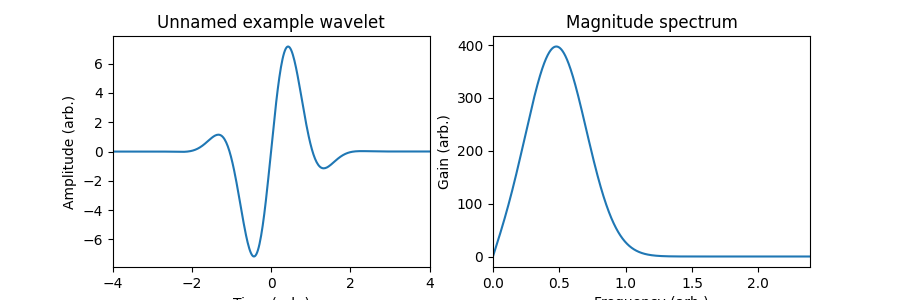The wavelet actually doesn’t need to be a bandpass, but rather somewhat bandpass-shaped. If it has a peak at a nonzero frequency and dips at least a little as you approach dc, it’s a useful wavelet. The wavelet $$\psi(t) = e^{-t^2} \cos(3 t)$$ is the real Morlet wavelet. As you can see from the value of its magnitude spectrum at 0 frequency, its dc bias is not exactly zero, but still looks reasonably like a bandpass. This is a very common wavelet, and the one we’ll be using for many examples.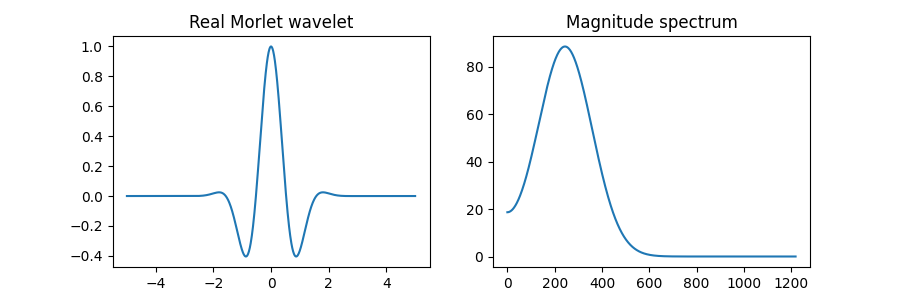Wavelets can also be complex-valued, such as in the complex Morlet wavelet: $$\psi(t) = \exp(3jt - t^2)$$.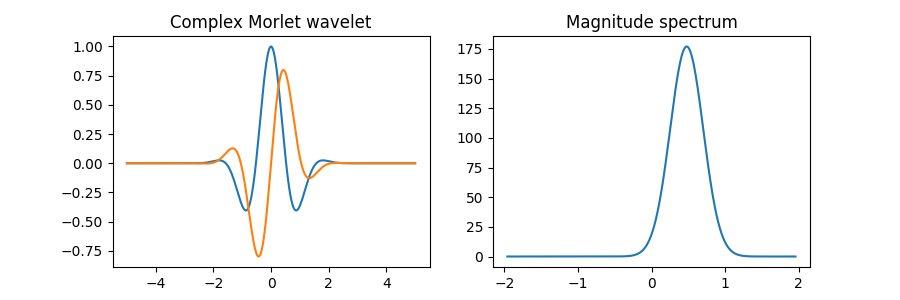[TODO: Vertical and horizontal scales may be wrong on the frequency-domain plots.]

As far as I can tell real wavelets are slightly more common than complex wavelets, although both are used. We’ll stick to the real Morlet wavelet and talk about other established wavelets in a later section.

### Time-shifting the mother wavelet

Given a square-integrable input signal $$f$$, consider the inner product of $$f$$ with $$\psi$$:

\begin{equation*} \langle f, \psi \rangle = \int_{-\infty}^{\infty} f(u) \psi(u)^* du \end{equation*}

To gain some intuition over what this inner product is doing, it “analyzes” $$f$$ with $$\psi$$ and produces a single complex number that gives us information about the similarity of $$f$$ and $$\psi$$. If $$f$$ has a lot of energy exactly where $$\psi$$ has energy, then $$\langle f, \psi \rangle$$ is likely to be higher in magnitude. If $$f$$ and $$\psi$$ don’t really line up much then the inner product will be close to zero.

However, because of $$\psi$$’s time-locality, this analysis only gives us information about a certain window of time. To gain more information about $$f$$, let’s shift the mother wavelet around in time $$t \in \mathbb{R}$$:

\begin{equation*} \psi_t(u) = \psi(u - t) \end{equation*}

We can then take the inner product $$\langle f, \psi_t \rangle$$ to analyze $$f$$ at any time. If we expand the inner product

\begin{equation*} \langle f, \psi_t \rangle = \int_{-\infty}^{\infty} f(u) \psi(u - t)^* du \end{equation*}

then sharp readers will notice that, as a function of $$t$$, $$\langle f, \psi_t \rangle$$ is just convolution of $$f$$ with the complex conjugate of the mother wavelet $$\psi$$. Since we are convolving $$f$$ with a wavelet, this is just a bandpass filter — nothing exciting yet.

### Scaling the mother wavelet

The mother wavelet is also frequency-local, so evaluating $$\langle f, \psi_t \rangle$$ for every $$t$$ only really gives us information about $$f$$ in one frequency band. Here is where the wavelet magic comes in. We scale the mother wavelet in time to change its frequency range:

\begin{equation*} \psi_{s,t}(u) = \psi\left( \frac{u - t}{s} \right) \end{equation*}

where $$s \neq 0$$ is the scaling factor and $$t$$ is the time shift applied after scaling. $$s > 1$$ expands or dilates the wavelet, $$0 < s < 1$$ compresses it, $$s < 0$$ performs time-reversal and scaling. In practice, negative scales $$s < 0$$ are often ignored. The full gamut of functions $$\psi_{s,t}$$ gives us the full set of daughter wavelets.

The scale multiplies the center frequency of the bandpass-shaped filter by a factor $$1/s$$. So when you hear high scale, think lower frequency, and vice versa.

It is generally desirable to have all daughter wavelets retain unit norm. From the properties of the norm, we multiply the daughter wavelet by the following corrective factor.

(Note that sometimes this factor is written as $$1/|s|$$ or omitted entirely, depending on the application.)

A property of the Fourier transform states that time-scaling a function around 0 also scales its Fourier transform. When a wavelet is scaled by $$s$$, its frequency response is scaled by $$1 / s$$. (Its amplitude also changes.) Thought of as a filter, scaling moves around its center frequency.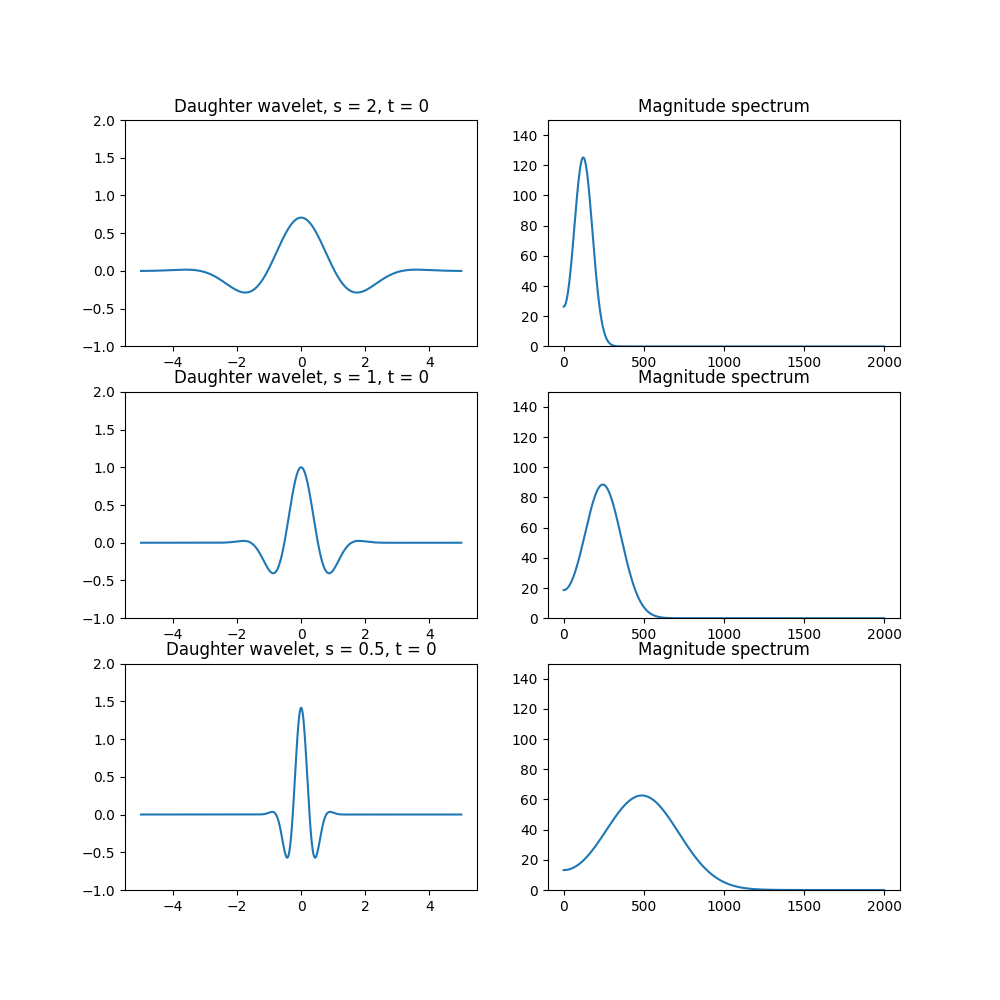Its bandwidth is also scaled by the same amount, so its Q remains constant. For this reason the CWT overlaps with some versions of the Constant-Q Transform, but alas CQT means different things depending on who you talk to.

In audio, it’s common to view filter frequency responses on a log-log scale, like in typical parametric equalizers. Here are a few frequency responses of selected Morlet wavelets with logarithmic frequency and decibel units: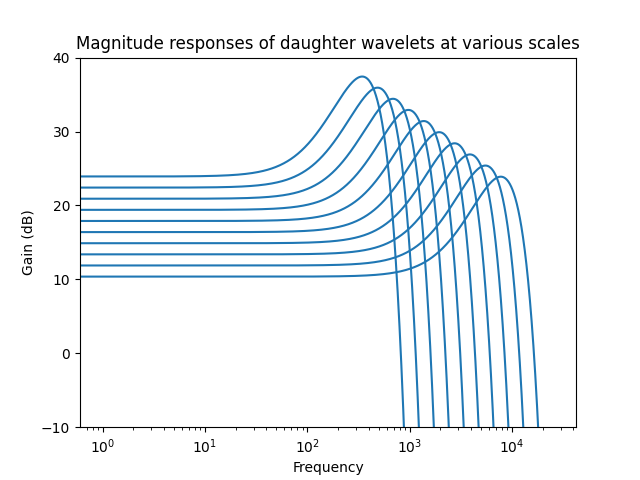As you can see, they are all formed by a rigid “diagonal” translation of the mother wavelet. The horizontal component of this translation is the result of frequency scaling, while the vertical component is just a change in gain.

### Definition of the CWT

We now have all the information we need to define the CWT. With the inner product and the daughter wavelets defined, the formula for expressing the transform is particularly terse.

While the Fourier transform turns a one-dimensional function of time into a one-dimensional function of frequency, the CWT produces a two-dimensional function, with the parameters of scale and time shift. There is no discretization here: $$\tilde{f}(s, t)$$ is defined for every $$s \neq 0$$ and every real $$t$$.

Being complex, $$\tilde{f}(s, t)$$ has both phase and magnitude. (If the signal and wavelet are real-valued, $$\tilde{f}(s, t)$$ is of course real, but it can be negative.) If we plot the magnitude $$|\tilde{f}(s, t)|$$ as a two-dimensional image with time on the horizontal axis and scale on the vertical axis, we produce something called the scalogram. The visual of the scalogram is extremely useful for understanding the CWT and its variants.

If we take this rectangular-windowed sine wave sweep: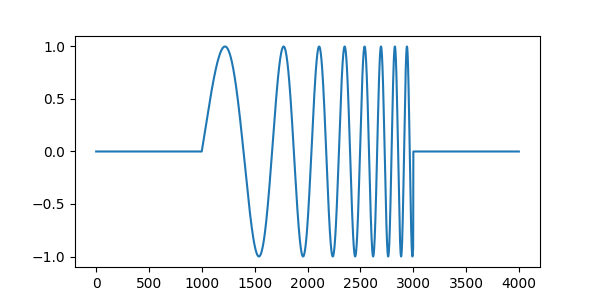and the real Morlet wavelet, we may view our first scalogram: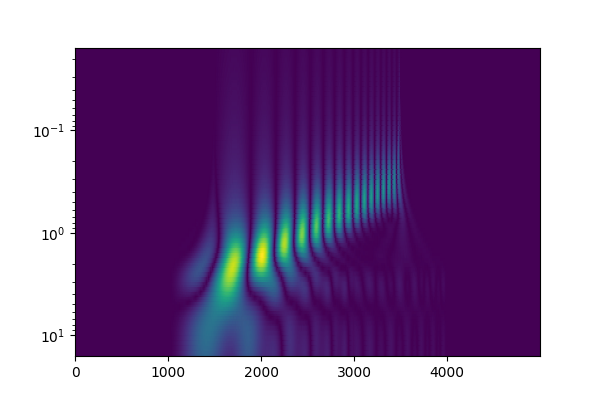[TODO: There might be windowing artifacts in this since I had to truncate the wavelet for practical computation.]

I’ve followed two common conventions in scalograms: a log scale for the vertical axis, and to place smaller scales (and therefore higher frequencies) towards the top.

You can see a diagonal line here that looks pretty close to straight, indicating that the frequency of the sine wave sweep I put in is exponential. We could have also learned that from a spectrogram. However, a typical spectrogram would show a continuous line; here the scalogram breaks up the sweep into a discrete set of “hot spots.” This scalogram’s time resolution divides the sine wave into individual pulses, while still being aware of its changing frequency. However, the time-frequency resolution situation is dependent on the choice of wavelet.

Keep in mind that scalograms, like spectrograms, typically depict only magnitude and not phase, so they don’t contain all the information that the CWT provides.

### Inverse Continuous Wavelet Transform

With the CWT established, musicians familiar with FFT processing via the phase vocoder are likely itching to start modifying and inverting the CWT to create scalogram-based effects. Not so fast, soldier.

My own introduction to time-frequency transforms was through FFT in computer music, and I imagine that’s likely the case for much of my audience. It was a rude awakening for me to learn that the Fourier transform is rather special, and in general not all time-frequency transforms are actually invertible in theory and practice. Many wavelet transform variants fail to be invertible at all.

The CWT, the primordial organism that every wavelet-like transform descends from, is in fact invertible. Sort of, kind of, sometimes. If I give you the CWT $$\tilde{f}$$ of a signal, the Inverse Continuous Wavelet Transform (ICWT) allows us to recover $$f$$. However, there are some important caveats:

• It is easy to come up with $$\tilde{f}$$ that do not have any inverse CWT. If I modify $$\tilde{f}$$ even a tiny amount, there may not be any $$f$$ that corresponds to it, because the CWT is highly redundant. This is in stark contrast to, say, the discrete Fourier transform, where any modification done in the Fourier domain will always have a valid inverse.

• Actually computing the ICWT is difficult in the general case. Some wavelets make it easier. [TODO: explain which ones]

• A constant bugbear in the design of FFT pitch shifters, etc. is the problem of phase coherence. In the case of the CWT, phases are even more unintuitive and difficult to work with than they are in FFT.

• If $$\psi$$ has a dc offset of 0, then the CWT can’t possibly capture any information about the dc offset of $$f$$, and the ICWT cannot recover it. This isn’t a huge problem for audio signals where dc offsets are generally considered a nuisance, but it’s worth noting to avoid confusion.

Overall, my impression from the literature is that the ICWT is not very common. The CWT is used most frequently for analysis and visualization, and not so much for processing.

Due to all the thorny problems involved, I won’t cover the ICWT in depth. However, we will later discuss an easily invertible variant of the CWT called Multiresolution Analysis.

## Discrete-Time Continuous Wavelet Transform

The Continuous Wavelet Transform, as described, is not actually implementable on a computer since takes an analog signal and produces an infinitely dense 2D plane of complex numbers. The CWT can be modified to operate on discrete-time signals, resulting in a discretized version of the CWT. Often this is just called the CWT in practical applications, other times it’s called the “Discrete-Time Continuous Wavelet Transform” or DT-CWT. I don’t need to point out how irritating the naming situation is, but unfortunately, the term “Discrete Wavelet Transform” typically refers to something different.

In the DT-CWT, the mother wavelet $$\psi$$ is still continuous-time and square-integrable with unit norm. The daughter wavelets are again produced by time-shifting and scaling, but after that they are sampled into discrete signals in $$\ell^2(\mathbb{Z})$$:

\begin{equation*} \psi_{s,t}[u] = \frac{1}{\sqrt{s}} \psi\left( \frac{u - t}{s} \right) \end{equation*}

This is of course the same formula as before, but I’ve used square brackets to indicate that $$\psi_{s,t}$$ now takes only integer values of $$u$$. Note that although the time parameter $$u$$ is discrete, $$s$$ and $$t$$ are still continuous.

Many (although not all) wavelets are non-bandlimited, so by picking values of the daughter wavelets at integer time points, we may introduce aliasing. However, we know that $$\psi$$ is bandpass-shaped, so there is some frequency that $$\psi$$ has negligible energy above. This lets us place a lower bound on how small $$s$$ can be. In particular, if $$\psi$$ has negligible energy above frequency $$F$$ then we only need $$s > F / \text{Nyquist}$$. There is no need for a specialized antialiasing mechanism: the wavelet itself already provides our lowpass!

The DT-CWT is pretty much identical to the CWT:

But $$\tilde{f}(s, t)$$ is still defined for all real $$s$$ and $$t$$, so this is still not fully “discrete.” We have to find some way to quantize $$s$$ and $$t$$.

### Quantizing frequency

Generally there’s not much use in really fine-grained shifts of $$t$$ that are less than a sample, so it’s okay to assume that $$t$$ is an integer.

In the case of $$s$$, a common approach is to use exponentially spaced scaling factors, such as $$s = 2^{k / d}$$ where $$k$$ is an integer index and $$d$$ is a number of divisions we care about per octave. For analysis of Western music, setting $$d$$ to a multiple of 12, such as 24 or 48, is a possible choice.

There is a natural lower limit to $$s$$, because at some point the daughter wavelets are so squashed that they have very little energy below Nyquist. As mentioned above, this bound is $$s > F / \text{Nyquist}$$ where the mother wavelet has little to no energy above $$F$$. There is nothing stopping you from using smaller $$s$$ values, but the discretized daughter wavelets will just be aliased garbage and won’t tell you anything that higher $$s$$ values don’t do.

In theory, $$s$$ has no upper limit. As it approaches infinity the daughter wavelets analyze lower and lower frequencies and approach dc. However, in all practical applications you have a lower bound on useful frequencies. In audio, this is usually the 20 Hz lower limit on human hearing.

Although it is common in applications to use exponentially spaced $$s$$ values, be aware that it isn’t a requirement. If you want, you can use linear frequencies, or mel frequencies, or Bark frequencies, or a small set of hand-picked frequencies (such as DTMF tones).

SciPy’s scipy.signal.cwt implements the DT-CWT where $$t$$ is set to all integer sample values, and the values of $$s$$ are specified as an arbitrary array. (See docs.) Notably, SciPy does not provide an icwt that takes us back to the time domain.

### Downsampling DT-CWT bands

To recap, we started by conceptualizing the CWT as an infinitely dense bank of bandpass filters on a continuous-time signal. With the DT-CWT we discretized the input signal, then we quantized the frequency bands, producing a finite bank of bandpass filters. At this point we have an algorithm with a straightforward computer implementation.

If you think about what happens for lower frequencies and larger values of $$s$$, the signal formed by convolving with the corresponding daughter wavelets has little high-frequency energy. If the mother wavelet has little to no energy above a frequency $$F$$, then the signal produced by bandpass-filtering the input at scale $$s$$ is effectively bandlimited at $$F / s$$. If $$F / s < \text{Nyquist} / 2$$ then we don’t lose much information by downsampling the signal by a factor of two. So, for $$s \geq 2 F / \text{Nyquist}$$ we only care about every other sample. As the signal is already quasi-bandlimited, the downsampling can be done by naively dropping every other sample; no additional filtering is required. Put another way, for suitably large $$s$$ our set of daughter wavelets can be reduced by only considering $$\psi_{s,t}$$ for even $$t$$.

In general, for scale $$s$$, the corresponding bandpassed signal can be downsampled by up to a factor of $$k$$, where $$k = \left\lfloor \frac{sF}{\text{Nyquist}} \right\rfloor$$. Since we compute the CWT through a stack of convolutions, we can reduce computational cost by just not computing the dropped samples in the first place.

Let’s consider a DT-CWT with 12 bands per octave where each $$s$$ value has been downsampled by the maximum allowable integer factor. If we place a dot at every value of $$s$$ and $$t$$ corresponding to a daughter wavelet $$\psi_{s,t}$$, we’ll get something lilke this: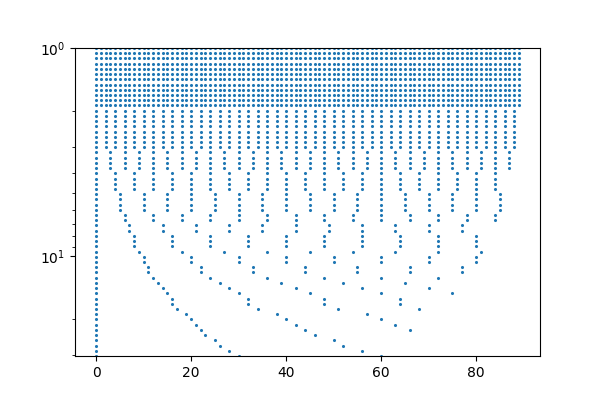Thus, the result of the DT-CWT is a single complex value at each of these dots. While somewhat pretty-looking (reminiscent of driving by orchards in NorCal), this downsampling scheme is not done in practice because the data structure needed to store these points is tough to work with. The pattern of dots is ultimately periodic along the time axis, but the period is very long.

I wrote that the downsampling can be done up to a factor of $$k$$, but it need not be exactly $$k$$. A common approach is to round the downsampling factors down to integer powers of 2.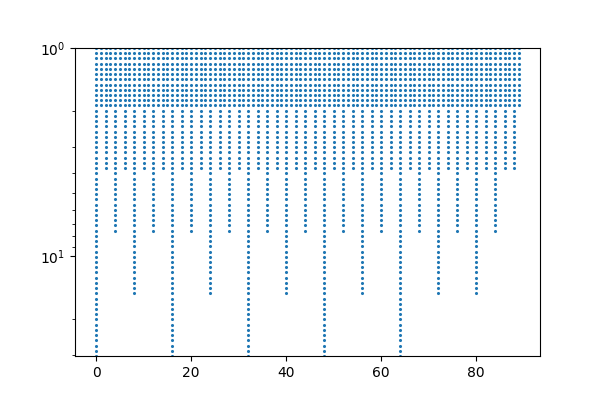In exchange for slightly suboptimal downsampling, we have something much easier to work with. The pattern of dots has a short period, which is simply the time distance between samples in the bottom octave. (We still get all these benefits even if the spacing of $$s$$ is not exponential.)

This variable-rate downsampling is more than just a shortcut to save space and kiloflops. It’s a fundamental component of our next topic:

## Multiresolution Analysis (Fast Wavelet Transform)

If you thought the DT-CWT sounds like a fairly intensive algorithm, you’d be right — as described, it’s a heavy operation. You may wonder if there is an equivalent of the Fast Fourier Transform for the DCWT.

There is in fact a Fast Wavelet Transform, but unlike the FFT, the Fast Wavelet Transform is not a fast implementation of the CWT, but something different. It’s closely related to the CWT, and it is lends itself to performance optimization much easier than the DT-CWT, but it’s different from the DCWT in crucial ways.

In particular, the FWT implements a constrained form of CWT called Multiresolution Analysis (MRA). MRA differs from CWT in several crucial aspects:

1. Invertibility: MRA has no redundancy and is easy to invert. You can alter the MRA coefficients and perform an inverse FWT, and there will be no problems synthesizing a modified signal. The implementations of the forward and inverse FWT are both simple to implement and computationally efficient.

2. Restrictions on wavelets: MRA only has these nice properties for a special subset of wavelets known as orthogonal wavelets. The Morlet wavelet is not orthogonal, but the Haar and Daubechies wavelets are, and we’ll see them in a bit.

3. Coarse frequency resolution: In MRA, the scale parameter is constrained to nonnegative integer powers of 2: $$s = 1, 2, 4, 8, \ldots$$.  In musical terms, the frequency resolution is one octave per band.

The first means that MRA is immediately useful for the creation of novel musical effects. The last, however, is a serious bummer for music processing, since you just don’t get much frequency resolution with the Fast Wavelet Transform. This doesn’t meant that MRA is musically useless, but I want to temper any hopes that it’s some kind of magic bullet for effect design.

This section introduces the MRA. Bear with me, as it’s going to look pretty different from everything we’ve talked about. It will connect back with the CWT, I promise.

### Analysis with MRA

I’m going to assume real wavelets here. Modifications are necessary for complex wavelets, albeit only minor ones.

To implement MRA, we are given a reconstruction lowpass filter with impulse response $$h[t]$$ where $$t \in \mathbb{Z}$$ Practical implementations require $$h$$ to be FIR; IIR lowpass filters are also of use since they can be truncated.

We can’t just pick any $$h$$; it has to satisfy certain special properties which will be shortly described. One is that the cutoff of $$h$$ should be half Nyquist, or one quarter of the sample rate. [TODO: note amplitude normalization.] There is no requirement that $$h[t]$$ is causal, meaning it may be nonzero for $$t < 0$$. In e.g. image processing there is no notion of “causality,” but in real-time audio this adds a complication, one that we’ll talk about later.

From the reconstruction lowpass filter we produce three other filters:

\begin{equation*} \begin{align*} h^*[t] &= h[-t] \, \text{(analysis lowpass filter)} \\ g[t] &= -(-1)^t h[-t] \, \text{(reconstruction highpass filter)} \\ g^*[t] &= g[-t] \, \text{(analysis highpass filter filter)} \\ \end{align*} \end{equation*}

Here’s what they look like: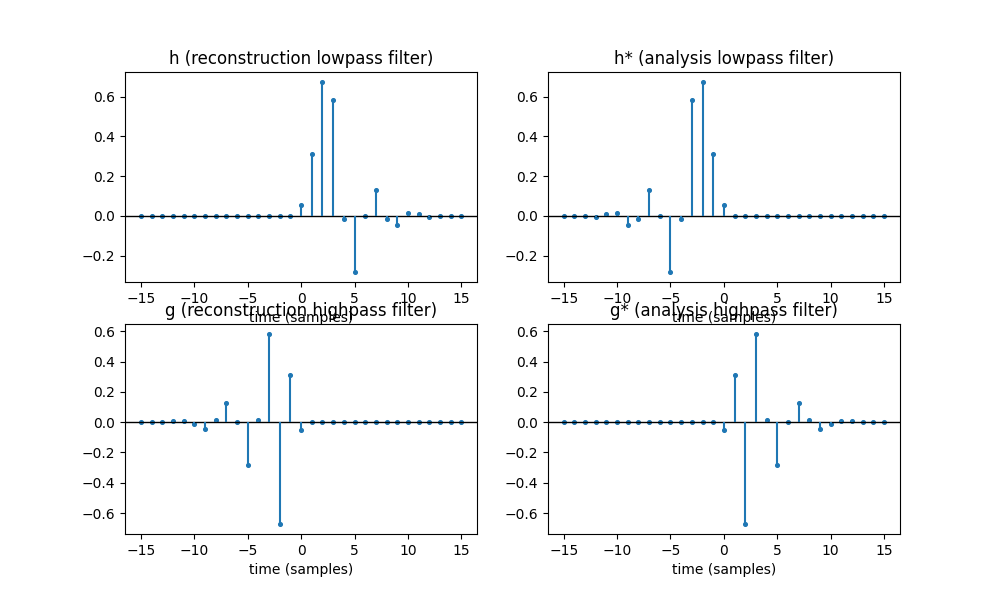[TOOD: fix crashing text]

$$h^*$$ is the time-reversal of the filter about $$t = 0$$. Filter nerds can verify that the convolution $$h * h^*$$ produces a zero phase lowpass filter whose magnitude spectrum is the square of the magnitude spectrum of $$h$$; this is the exact same principle as that of forward-backward filtering. (The superscript $$^*$$ is not quite the complex conjugate but rather the adjoint of the filter, which I don’t have the space to describe. Don’t confuse it with the centered $$*$$, which is plain old convolution.)

$$g$$ time-reverses $$h$$ and flips the sign of every other coefficient, and $$g^*$$ in turn time-reverses $$g$$. These are highpass filters: multiplying (modulating) a signal with a sine wave at Nyquist produces a spectral inversion. Again $$g * g^*$$ is zero phase, and its magnitude spectrum is the square of $$g$$’s magnitude spectrum.

The filters I’ve plotted above in this example are rather special ones, with $$h$$ being derived from the 8th-order Daubechies wavelet. These are FIR filters and they vanish to zero outside of the range I’ve plotted. Also, noting the ranges on the X-axes, we have $$h[t] = 0$$ for $$t < 0$$, so $$h$$ and $$g^*$$ are causal; this is not necessarily true of all filters. We can plot their magnitude responses on a linear-linear scale to confirm that these are indeed low and highpass filters whose cutoffs are half Nyquist: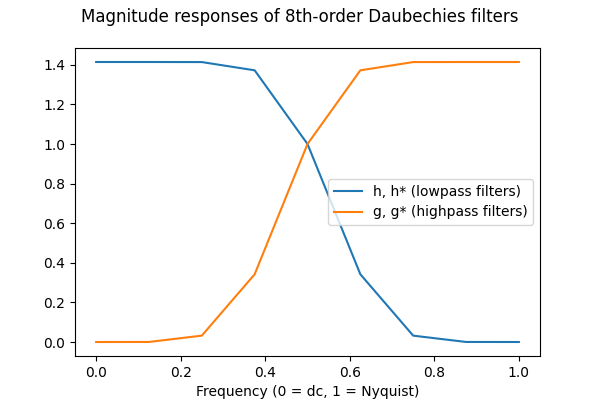$$g$$ and $$g^*$$ have the same magnitude responses and so do $$h$$ and $$h^*$$; between each adjoint pair the phase responses (not shown) are sign flips of each other.

We define the linear (but not time-invariant!) operators $$S_\Downarrow$$ and $$S_\Uparrow$$. $$S_\Downarrow$$ downsamples a signal by 2x by discarding every other sample: $$(S_\Downarrow f)[t] = f[2t]$$. $$S_\Uparrow$$ upsamples a signal 2x by inserting zeros: $$(S_\Uparrow f)[2t] = f[t]$$ and $$(S_\Uparrow f)[2t + 1] = 0$$. In the frequency domain, $$S_\Downarrow$$ folds the top half of the digital signal down to the top half, and $$S_\Uparrow$$ mirrors the entire spectrum around half Nyquist.

We are now ready to define the Fast Wavelet Transform:

I know you’re looking for a block diagram, so here it is, topping out at $$J = 3$$: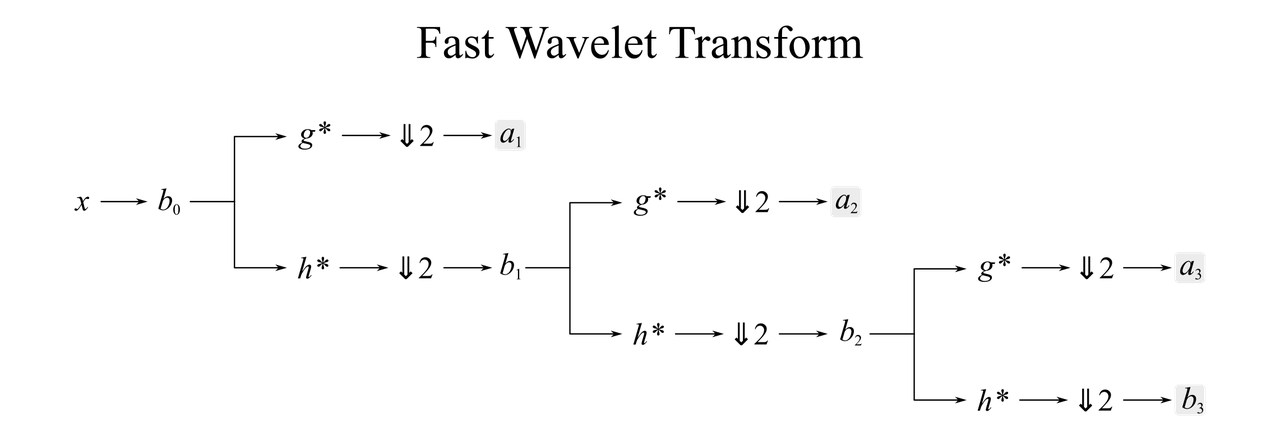The output signals are outlined with gray boxes. Note that the $$a_\cdot$$ and $$b_\cdot$$ steps are just variable names, not actual filters or processing steps.

The downsampling operator naturally introduces aliasing, but since $$h^*$$ is a lowpass, it acts as an antialiasing filter by attenuating the high frequencies. Complementarily, $$g^*$$ filters out the low frequencies, and the downsampling operator mirrors these high frequencies around half Nyquist.

If we only want to use MRA to analyze the signal, then we can just take our downsampled signals and be done with it. But a magical property of the MRA states that, under certain conditions, it is possible to use these analysis signals to recover $$u^0$$.

Block diagram block diagram block diagram:In plain English, we take the lowpass/highpass pair, upsample them, and convolve them with the reconstruction lowpass and highpass filters. Then we add them together to try to reconstruct the highpass signal.

I wrote that we try to reconstruct $$b_0$$. In reality, $$h[t]$$ must be designed in the first place so that perfect reconstruction is possible. In practice, someone implementing an MRA will know a priori that $$h[t]$$ is suited for this purpose. The takeaway is that you can’t plug random lowpass filters into MRA and get perfect reconstruction.

### Implementation details

This algorithm is almost implementable right out of the block diagram, but we first need to discuss a minor optimization and a major caveat.

[TODO: polyphase filtering, latency compensation in non-causal filters]

### Connecting MRA to the CWT, part 1: the intuition

It is entirely understandable if you are having trouble understanding the relationship to wavelets here. That was the case for me when I first looked at the FWT algorithm. However, as promised, there is a deep connection between these two transforms. The MRA-CWT linkage gets a little technical, and we’ll see a more precise treatment in the next section, but I want to set it up with a visual explanation first.

The recursive downsampling scheme results in multiple streams at different sampling rates, all of which are power-of-two divisions of the original signal’s sample rate. If we place a dot at the time of every sample, and write the different streams as rows, we get a familiar diagram:

[TODO: MRA dots diagram]

If you guessed that the MRA values are in fact inner products produced by the DT-CWT at power-of-two scales, you win a point:

This is quite beautiful, but why this is the case isn’t obvious. To gain some intuition, we’ll examine what the MRA is doing in a filter theory sense. As a jumping-off point, we’ll start with the signal $$a_2$$ produced by the FWT. $$a_2$$ is the result of the following operations on the input signal:

1. Lowpass filter with cutoff $$f_N/2$$ and downsample by a factor of 2.

2. Highpass filter with cutoff $$f_N/4$$ and downsample again by a factor of 2. (Here $$f_N$$ is the Nyquist frequency of the original signal prior to downsampling. Relative to the current signal’s sample rate, the cutoffs of these filters are always half Nyquist; a common cuss word for these is “half-band filter.”)

If we ignore the downsampling operations for a moment, we find that this is a highpass filter followed by a lowpass filter, which is a bandpass that roughly isolates the frequency content in the range $$[f_N / 4, f_s / 2]$$. What about $$a_3$$?

1. Lowpass filter with cutoff $$f_N/2$$ and downsample by a factor of 2.

2. Lowpass filter with cutoff $$f_N/4$$ and downsample by a factor of 2.

3. Highpass filter with cutoff $$f_N/8$$ and downsample by a factor of 2.

Again, if the downsampling operations are ignored, this is a bandpass filter that isolates the range $$[f_N / 8, f_s / 4]$$. In general, we may conclude that in the absence of downsampling, the $$a_m$$ signals for $$m > 1$$ are the result of a bandpass filter with a rough passband of $$[2^{-m - 1} f_s, 2^{-m} f_s]$$.

The connection to the CWT should start to feel closer now that bandpass filters have shown up, but we aren’t quite home free yet; we still have those pesky downsampling operations, which being non-time-invariant are not guaranteed to commute with other operations. It turns out that they do commute approximately, and we can conceptually move all the downsampling to the end: 

1. Bandpass filter to the range $$[2^{-m - 1} f_N, 2^{-m} f_N]$$.

2. Downsample by a factor of $$2^{-m}$$. As the signal only has significant energy up to $$2^{-m} f_N$$, there’s no aliasing.

3. Downsample by a factor of 2. This folds the upper half of the spectrum and spectrally inverts it to the range $$[0, 2^{-m - 1} f_N]$$.

So the MRA really does have bandpass filter-like functionality, and in step 2 it downsamples out redundant information as we discussed in the DT-CWT. Step 3 isn’t something we’ve seen before — it further compresses the DCWT band with an additional downsampling stage. As this just amounts to leaving out half the dots on the DCWT, we are still technically evaluating the DCWT, just at fewer locations. The fact that we are able to omit half the points without information loss is specific to the shape of the bandpass filter; if its lower band edge was any lower than $$2^{-m - 1} f_N$$ then the signal crashes into itself. (This may be the case if the wavelet is not orthogonal, such as the Morlet wavelet.)

The impulse response of the bandpass filter is of course a daughter wavelet. With the information we have, we can compute the daughter wavelet itself: it is a cascade of $$m$$ analysis lowpass filters at increasing power-of-two scales, followed by one last highpass filter at scale $$m$$. Since all daughter wavelets are related by scaling and time-shifting, we can compute the mother wavelet to arbitrary time precision by increasing $$m$$ as high as we want.

I didn’t mention the final $$b_J$$ signal at the bottom of the FWT. All you need to know for intuition is that this signal’s chain is just lowpasses, and therefore it isn’t actually part of the DT-CWT. To properly describe it we need yet another new concept (sorry), that of the scaling function — and we’ll leave that for the nerds to peruse in the next section.

### Connecting MRA to the CWT, part 2: the math

This section is optional. [TODO: This section also probably has some mistakes.]

Welcome, nerds. A scaling function is denoted $$\phi(t)$$, a square-integrable function $$\mathbb{R} \rightarrow \mathbb{C}$$. Like a mother wavelet, it has both time- and frequency-locality. Unlike a wavelet, $$\phi(t)$$ has a dc value (integral) of 1 instead of 0, so the frequency locality is around dc. The scaling function is a lowpass rather than a bandpass; there is no specific requirement of what its cutoff is.

For convenience, we define a dilation operator $$D$$, which stretches out a function by factor of 2 and retains its norm:

\begin{equation*} Df(u) = \frac{1}{\sqrt{2}} D(u / 2) \end{equation*}

and a time-shift operator, which displaces the function in time by an integer shift:

\begin{equation*} T^nf(u) = f(u - n) \end{equation*}

Scaling functions do not exist for general wavelets as far as I understand; they are only used in conjunction with orthogonal wavelets in the context of MRA. (Many wavelet resources fail to make this clear, and it tripped me up a lot.) An orthogonal wavelet has exactly one associated scaling function, and a scaling function has exactly one asssociated orthogonal wavelet if it satisfies a property called the two-scale relation:

\begin{equation*} \phi(u) = \sum_{t = -\infty}^{\infty} h[t] \phi(u - t) = h * D\phi \end{equation*}

where $$h[t]$$ is an integer-indexed sequence of real numbers. In English, the dilation of $$\phi$$ is a linear combination of copies of the original $$\phi$$ translated by integer time shifts. The coefficients $$h[t]$$ are precisely the reconstruction lowpass filter used in the FWT. Note that $$h * \phi_{2,0}$$ is a mild abuse of notation because $$h$$ is a discrete signal and $$\phi_{}$$ is a continuous signal; to make this formally valid imagine that $$h$$ is realized as train of Dirac delta functions at integer time values (and therefore its Fourier transform is periodic).

Some wavelet schemes start with $$h[t]$$ and use that to define the scaling function. Others go in the opposite direction, starting with $$\phi(t)$$ and deriving $$h[t]$$:

\begin{equation*} h[n] = \int_{-\infty}^\infty D\phi(t)^* \phi(t - n) = \langle T^n\phi, D\phi \rangle \end{equation*}

In either case, the final piece of the puzzle, which you have likely been anticipating if you made it this far, is to connect $$\phi(t)$$ to the actual mother wavelet $$\psi(t)$$ ([Mallat1999], Theorem 7.3):

\begin{equation*} D\psi = g * \phi \end{equation*}

[TODO: Above formula may be sligthly incorrect, check normalization factor.]

Thus the mother wavelet is the combination of the lowpass filter $$\phi$$ and the highpass filter $$g$$, squashed by a factor of two. That a lowpass and highpass compose to form a bandpass should square with our intuition about wavelets.

With these three equations, we can convert among MRA filter coefficients, wavelets, and scaling functions. Converting between the three is not guaranteed to be simple — sometimes $$h$$ can be derived but $$\phi$$ and $$\psi$$ lack a closed form, and other times we’re given $$\psi$$ or $$\phi$$ and we need numerical methods to find $$h$$. In all cases, remember that these conversions only make sense if the following equivalent statements are true: the wavelet is orthogonal, the scaling function satisfies the two-scale relation, and the filter admits perfect reconstruction.

Complicated? Sure. Fortunately, these aren’t tools you’ll need much for typical use and implementation of FWT. In many cases, you’re just given $$h$$. I’ve done this excursion into the math because I wanted an explanation that personally makes sense to me.

## Specific wavelets

So far, we’ve only seen two actual wavelets: the real and complex Morlet wavelets for the CWT, and one of the Daubechies wavelets for the FWT. Let’s go over a few other common one-dimensional wavelets. Normalization factors are usually not shown for simplicity.

### Morlet wavelet (Gabor wavelet)

[Morlet wavelet diagram]

Real or complex, infinite support, not orthogonal.

The Morlet wavelet is likely the most commonly used wavelet for the CWT. It has both real and complex versions. The real version is formed by enveloping a cosine wave with a Gaussian:

\begin{equation*} \psi(t) = e^{-t^2} \cos(\omega t) \end{equation*}

where $$\omega$$ determines the Q factor of the filter, with higher $$\omega$$ resulting in higher Q. The complex version replaces the cosine wave with a complex sinusoid:

\begin{equation*} \psi(t) = e^{-t^2} e^{j \omega t} \end{equation*}

### Haar wavelet

[Haar wavelet & scaling function diagram]

Real, finite support, orthogonal.

The Haar wavelet predates the wavelet transform by decades, when it formed the basis of the Haar transform. It is a rectangular-shaped “wiggle:”

\begin{equation*} \phi(t) = \begin{cases} 1 & 0 \leq t \leq \frac{1}{2} \\ -1 & \frac{1}{2} < t \leq 1 \\ 0 & \text{otherwise} \\ \end{cases} \end{equation*}

Its scaling function is even simpler, a rectangular bump:

\begin{equation*} \phi(t) = \begin{cases} 1 & 0 \leq t \leq 1 \\ 0 & \text{otherwise} \\ \end{cases} \end{equation*}

It can be confirmed visually that $$\phi(2t) = (\phi(t) + \phi(t - 1)) / 2$$, so the filter coefficients are $$h = \frac{1}{\sqrt{2}}[1,\,1]$$.

### Shannon wavelet

[Shannon wavelet & scaling function diagram]

Real, infinite support, orthogonal.

The Shannon wavelet is the wavelet answer to the ideal filter, or sinc filter. It’s only really useful as a theoretical ideal, not as a practical wavelet. It is easiest to define with its scaling function:

\begin{equation*} \phi(t) = \frac{\sin(\pi t)}{\pi t} = \text{sinc}(t) \end{equation*}

### Daubechies wavelets

[Daubechies wavelets & scaling functions diagram]

Real, finite support, orthogonal.

The Daubechies wavelets are common choices for MRA. The $$n$$ th-order Daubechies wavelet’s filter has length $$2n$$; the case $$n = 1$$ is the Haar wavelet.

The Daubechies wavelets are most easily specified as filter coefficients, but even they requires a fairly intricate numerical algorithm. Fortunately, you likely don’t need to implement that – SciPy has scipy.signal.daub, which gives you the FIR filter coefficients. $$\phi(t)$$ and $$\psi(t)$$ have no closed form, and generally have a complex fractal structure. They look pretty trippy, especially for naturally occuring mathematical functions.

### Mexican hat wavelet (Ricker wavelet)

[Mexican hat wavelet diagram]

Real or complex, infinite support, not orthogonal.

The Mexican hat wavelet (more boringly, the Ricker wavelet) is real:

\begin{equation*} \psi(t) = (1 - t^2) e^{-t^2} \end{equation*}

### Others

Other orthogonal wavelets include the Meyer wavelet, symlets, and coiflets. If you aren’t constrained by orthogonality, then practically any function with reasonable time and frequency locality can be used as a wavelet. For example, for audio some have used the gammatone or gammachirp functions as wavelets.

## Conclusion

Phew! At over 8,000 words, this is by far the longest and most ambitious work of writing I’ve ever done. (I had to switch the entire site from MathJax to KaTeX because the math equations became incredibly slow to load.)

If you made it through all that or even skimmed through it, then congratulations. Here’s some bullet points on the most important takeaways about the CWT and the MRA.

To be frank, when I started seriously investigating wavelets for audio applications, I was a little disappointed — the CWT and MRA aren’t as musical as I hoped. The theory of wavelets is beautiful, but in the audio space STFT almost always gets the job done.

However, when I dug deeper into the numerous wavelet transform variants developed over the years, I started finding more unusual transforms that seem to have more musical potential. I won’t divulge what exactly they are yet, as I’m currently experimenting with them for future projects. Stay tuned!

## Acknowledgements

Thanks to Julius Smith and R. Tyler McLaughlin for feedback and comments.